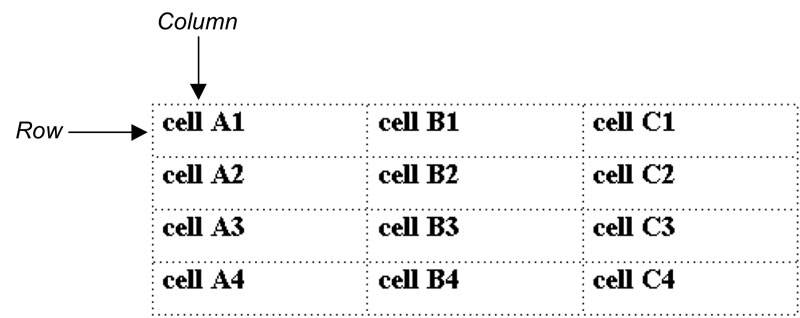# Calculating in Tables in Microsoft Word

Word lets you perform calculations on numerical table data and display the results in the table. For example, you can add a row or column of numbers, or you can add, subtract, multiply, or divide the contents of individual cells. Entering calculations into a table, instead of typing in the results, ensures that any changes you make to the table’s data are automatically reflected in the calculated results whenever you update the table.

We provide MS Word training classes in Los Angeles, and onsite classes countrywide.

Word uses the location, or cell reference, of specific cells in the table to perform calculations on their numerical data. In Word tables, columns are identified as A, B, C, and so on from left to right, and rows are identified as 1, 2, 3, and so on from top to bottom. A cell is identified by the letter and number of the column/row intersection at which it appears, as illustrated in table below. For example, the cell reference of the cell located in the second column and second row of a table is B2.#### Using AutoSum

When you insert an AutoSum field into a cell, the sum of the numbers in the column above the cell or in the row to its left is automatically calculated. This enables you to add a row or column of numbers quickly. If the column above and the row to the left of the AutoSum cell both contain numerical data, the sum of the numbers in the column is calculated.

If a blank cell appears in the summed column or row, AutoSum calculates only the numbers in the cells after the blank cell, not the entire row or column. You can type zeros in blank cells to have AutoSum calculate the entire row or column. If you change the value of one of the summed numbers, you can update the AutoSum field to display the new results.

#### Method

###### To insert an AutoSum field:
1. Place the insertion point in the desired cell
2. From the Layout tab, in the Table Tools tab, select the Formula button
3. ###### Type one of the following:

=SUM(ABOVE) adds the numbers in the column above the cell you’re in.
=SUM(LEFT) adds the numbers in the row to the left of the cell you’re in.
=SUM(BELOW) adds the numbers in the column below the cell you’re in.
=SUM(RIGHT) adds the numbers in the row to the right of the cell you’re in.

###### To update AutoSum fields:
1. Select the table to update all AutoSum fields. or
2. Select the AutoSum field cell you want to update.
3. Right Mouse click and Select Update Field Or Press F9

#### Group Word training

Through our network of local trainers we deliver onsite Word training right across the country. Read a sample of Word training testimonials or obtain a quotation for onsite Word training.